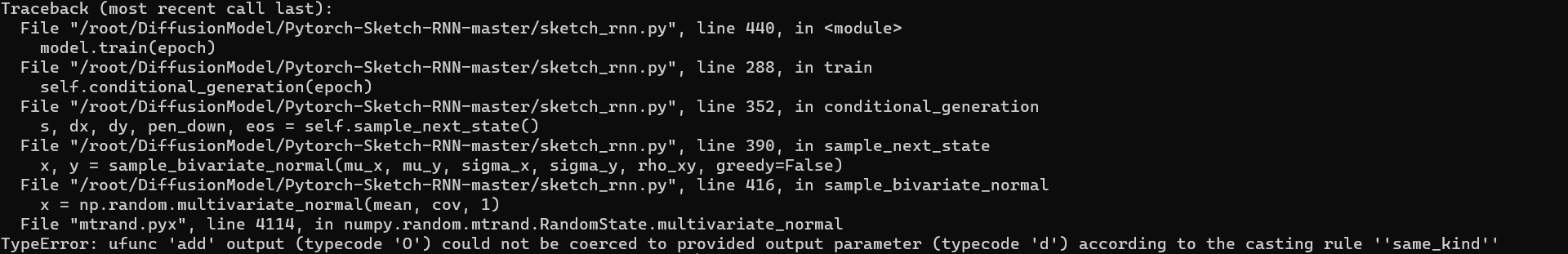WHCSRL 技术网

# 解决multivariate_normal中：output parameter (typecode ‘d‘) according to the casting rule ‘‘same_kind‘‘

sketch rnn

## 问题描述：

`完整报错：`

`` File "/root/DiffusionModel/Pytorch-Sketch-RNN-master/sketch_rnn.py", line 416, in sample_bivariate_normal    x = np.random.multivariate_normal(mean, cov, 1)  File "mtrand.pyx", line 4114, in numpy.random.mtrand.RandomState.multivariate_normalTypeError: ufunc 'add' output (typecode 'O') could not be coerced to provided output parameter (typecode 'd') according to the casting rule ''same_kind''````def sample_bivariate_normal(mu_x, mu_y, sigma_x, sigma_y, rho_xy, greedy=False):     if greedy:        return mu_x, mu_y    mean = [mu_x, mu_y]    sigma_x *= np.sqrt(hp.temperature)    sigma_y *= np.sqrt(hp.temperature)    cov = [[sigma_x * sigma_x, rho_xy * sigma_x * sigma_y],            [rho_xy * sigma_x * sigma_y, sigma_y * sigma_y]]     x = np.random.multivariate_normal(mean, cov, 1)    return x, x``

``x = np.random.multivariate_normal(mean, cov, 1)``

## 原因分析：

`np.random.multivariate_normal函数中使用了np.add操作，所以找到的资料大多是在np.add()中添加参数casting='unsafe'`

## 解决方案：

``def sample_bivariate_normal(mu_x, mu_y, sigma_x, sigma_y, rho_xy, greedy=False):     mu_x = mu_x.item()    mu_y = mu_y.item()    sigma_x = sigma_x.item()    sigma_y = sigma_y.item()     if greedy:        return mu_x, mu_y    mean = [mu_x, mu_y]    sigma_x *= np.sqrt(hp.temperature)    sigma_y *= np.sqrt(hp.temperature)    cov = [[sigma_x * sigma_x, rho_xy * sigma_x * sigma_y],            [rho_xy * sigma_x * sigma_y, sigma_y * sigma_y]]     x = np.random.multivariate_normal(mean, cov, 1)    return x, x``